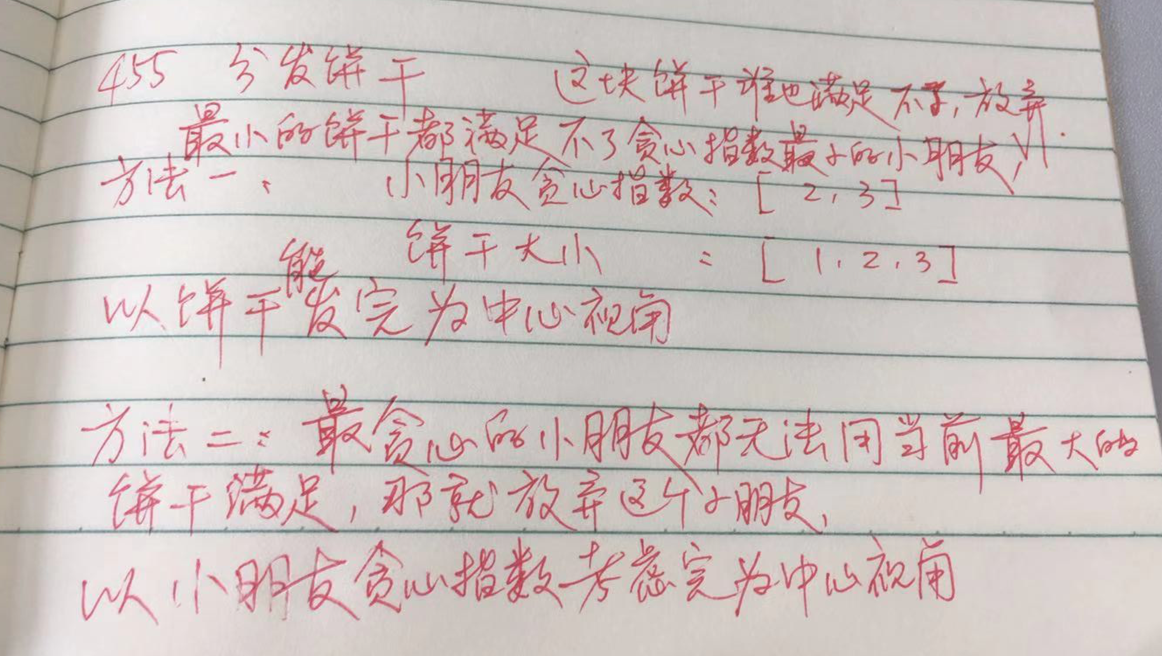# 「力扣」第 455 题：分发饼干

## 贪心算法（两个方向） + 优先队列（Python 代码）### 方法一：尽量用小的饼干满足贪心指数小的小朋友

Python 代码：

class Solution:
def findContentChildren(self, g: List[int], s: List[int]) -> int:
g.sort()
s.sort()

gi = 0
si = 0
res = 0

while gi < len(g) and si < len(s):
if s[si] >= g[gi]:
si += 1
gi += 1
res += 1
else:
si += 1
return res

Python 代码：

import heapq

class Solution:
def findContentChildren(self, g: List[int], s: List[int]) -> int:

heapq.heapify(g)
heapq.heapify(s)

res = 0
# 如果小的饼干满足不了贪心指数最小的小朋友，就放弃这个饼干
while g and s:
if s >= g:
heapq.heappop(g)
heapq.heappop(s)
res += 1
else:
heapq.heappop(s)
return res

### 方法二：尽量用大的饼干满足贪心指数大的小朋友

Python 代码：

class Solution:
def findContentChildren(self, g: List[int], s: List[int]) -> int:
g.sort(reverse=True)
s.sort(reverse=True)

gi = 0
si = 0
res = 0

while gi < len(g) and si < len(s):
if s[si] >= g[gi]:
si += 1
gi += 1
res += 1
else:
gi += 1
return res


Python 代码：

class Solution:
def findContentChildren(self, g: List[int], s: List[int]) -> int:
g = [(-num, num) for num in g]
s = [(-num, num) for num in s]
heapq.heapify(g)
heapq.heapify(s)

# 如果最大的饼干都满足不了胃口最大的小朋友，就放弃这个小朋友
res = 0
while g and s:
if s >= g:
heapq.heappop(g)
heapq.heappop(s)
res += 1
else:
heapq.heappop(g)
return res

上一篇「力扣」第 1081 题：不同字符的最小子序列
「力扣」第 1081 题：不同字符的最小子序列题解地址：贪心算法 + 栈、位掩码（Python 代码、Java 代码）。 说明：文本首发在力扣的题解版块，更新也会在第 1 时间在上面的网站中更新，这篇文章只是上面的文章的一个快照，您可以点击
2018-07-08「力扣」第 452 题：用最少数量的箭引爆气球
「力扣」第 452 题：用最少数量的箭引爆气球题解地址：贪心算法（Python 代码、Java 代码）。 说明：文本首发在力扣的题解版块，更新也会在第 1 时间在上面的网站中更新，这篇文章只是上面的文章的一个快照，您可以点击上面的链接看到其
2018-07-06
目录